### Example 84.6 Comparing Two Survival Distributions with a Log-Rank Test

This example requests a log-rank test that compares two survival distributions for the treatment effect (Jennison and Turnbull 2000, pp. 77–79; Whitehead 1997, pp. 36–39).

A clinic is studying the effect of a new cancer treatment. The study consists of mice exposed to a carcinogen and randomized to either the control group or the treatment group. The event of interest is the death from cancer induced by the carcinogen, and the response is the time from randomization to death.

Following the derivations in the section Test for Two Survival Distributions with a Log-Rank Test in the chapter The SEQDESIGN Procedure, the hypothesis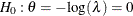with an alternative hypothesis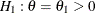can be used, where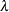is the hazard ratio between the treatment group and control group.

Suppose that from past experience, the median survival time for the control group is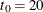weeks, and the study would like to detect a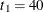weeks median survival time with a 80% power in the trial. Assuming exponential survival functions for the two groups, the hazard rates can be computed from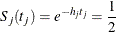where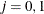.

Thus, with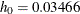and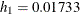, the hazard ratio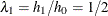and the alternative hypothesis is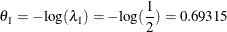The following statements invoke the SEQDESIGN procedure and request a four-stage group sequential design for normally distributed data. The design uses a one-sided alternative hypothesis with early stopping to reject and to accept the null hypothesis. Whitehead’s triangular method is used to derive the boundaries.

ods graphics on;
proc seqdesign altref=0.69315
boundaryscale=score
;
nstages=4
boundarykey=alpha
alt=upper  stop=both
beta=0.20;
samplesize model=twosamplesurvival
( nullhazard=0.03466
accrate=10);
run;
ods graphics off;


A Whitehead method creates boundaries that approximately satisfy the Type I and Type II error probability level specification. The BOUNDARYKEY=ALPHA option is used to adjust the boundary value at the last stage and to meet the specified Type I probability level.

The specified ACCRATE=10 option indicates that 10 mice will be accrued each week and the resulting minimum and maximum accrual times are displayed. With the BOUNDARYSCALE=SCORE option, the procedure displays the output boundaries with the score statistics.

The Design Information table in Output 84.6.1 displays design specifications and derived statistics.

The SEQDESIGN Procedure

Statistic Distribution Normal
Boundary Scale Score
Alternative Hypothesis Upper
Early Stop Accept/Reject Null
Boundary Key Alpha
Alternative Reference 0.69315
Number of Stages 4
Alpha 0.05
Beta 0.20044
Power 0.79956
Max Information (Percent of Fixed Sample) 129.9894
Max Information 16.70624
Null Ref ASN (Percent of Fixed Sample) 62.6302
Alt Ref ASN (Percent of Fixed Sample) 74.00064

The Boundary Information table in Output 84.6.2 displays the information level, alternative reference, and boundary values at each stage.

Output 84.6.2: Boundary Information

Boundary Information (Score Scale)
Null Reference = 0
_Stage_   Alternative Boundary Values
Information Level Reference Upper
Proportion Actual Events Upper Beta Alpha
1 0.2500 4.176561 16.70624 2.89498 -0.95755 4.78773
2 0.5000 8.353122 33.41249 5.78997 1.91509 5.74527
3 0.7500 12.52968 50.11873 8.68495 4.78773 6.70282
4 1.0000 16.70624 66.82498 11.57993 7.81296 7.81296

With ODS Graphics enabled, a detailed boundary plot with the rejection and acceptance regions is displayed, as shown in Output 84.6.3.

Output 84.6.3: Boundary Plot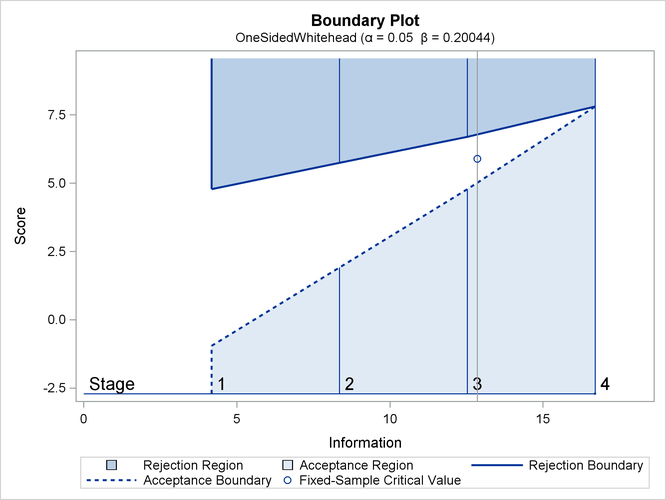With the MODEL=TWOSAMPLESURVIVAL option in the SAMPLESIZE statement, the Sample Size Summary table in Output 84.6.4 displays the parameters for the sample size computation.

Output 84.6.4: Required Sample Size Summary

Sample Size Summary
Test Two-Sample Survival
Null Hazard Rate 0.03466
Hazard Rate (Group A) 0.01733
Hazard Rate (Group B) 0.03466
Hazard Ratio 0.499999
log(Hazard Ratio) -0.69315
Reference Hazards Alt Ref
Accrual Uniform
Accrual Rate 10
Min Accrual Time 6.682498
Min Sample Size 66.82498
Max Accrual Time 25.401
Max Sample Size 254.01
Max Number of Events 66.82498

With a minimum accrual time of 6.6825 weeks and a maximum accrual time of 25.401 weeks, an accrual time of 20 weeks is used in the study.

The Numbers of Events table in Output 84.6.5 displays the required number of events for the group sequential clinical trial.

Output 84.6.5: Required Numbers of Events

Numbers of Events (D)
Two-Sample Log-Rank Test
_Stage_ D Information
1 16.71 4.1766
2 33.41 8.3531
3 50.12 12.5297
4 66.82 16.7062

The following statements invoke the SEQDESIGN procedure and provide more detailed sample size information:

proc seqdesign altref=0.69315
boundaryscale=score
;
nstages=4
boundarykey=alpha
alt=upper
stop=both
beta=0.20;
samplesize model=twosamplesurvival
( nullhazard=0.03466
accrate=10 acctime=20);
ods output Boundary=Bnd_Surv;
run;


The ODS OUTPUT statement with the BOUNDARY=BND_SURV option creates an output data set named BND_SURV which contains the resulting boundary information for the subsequent sequential tests.

With an accrual time of 20 weeks, the Sample Size Summary table in Output 84.6.6 displays the follow-up time for the trial.

Output 84.6.6: Required Sample Size Summary

The SEQDESIGN Procedure

Sample Size Summary
Test Two-Sample Survival
Null Hazard Rate 0.03466
Hazard Rate (Group A) 0.01733
Hazard Rate (Group B) 0.03466
Hazard Ratio 0.499999
log(Hazard Ratio) -0.69315
Reference Hazards Alt Ref
Accrual Uniform
Accrual Rate 10
Accrual Time 20
Follow-up Time 6.47422
Total Time 26.47422
Max Number of Events 66.82498
Max Sample Size 200
Expected Sample Size (Null Ref) 161.5937
Expected Sample Size (Alt Ref) 172.4689

The Numbers of Events and Sample Sizes table in Output 84.6.7 displays the required sample sizes for the group sequential clinical trial.

Output 84.6.7: Numbers of Events and Sample Sizes

Numbers of Events (D) and Sample Sizes (N)
Two-Sample Log-Rank Test
_Stage_ Fractional Time Ceiling Time
D D(Grp 1) D(Grp 2) Time N N(Grp 1) N(Grp 2) Information D D(Grp 1) D(Grp 2) Time N N(Grp 1) N(Grp 2) Information
1 16.71 5.82 10.89 11.9866 119.87 59.93 59.93 4.1766 16.74 5.83 10.91 12 120.00 60.00 60.00 4.1854
2 33.41 11.84 21.57 17.3584 173.58 86.79 86.79 8.3531 35.73 12.68 23.04 18 180.00 90.00 90.00 8.9322
3 50.12 18.01 32.11 21.7479 200.00 100.00 100.00 12.5297 51.07 18.37 32.70 22 200.00 100.00 100.00 12.7667
4 66.82 24.46 42.37 26.4742 200.00 100.00 100.00 16.7062 68.55 25.14 43.41 27 200.00 100.00 100.00 17.1377

Thus the study will perform three interim analyses after 12, 18, and 22 weeks and a final analysis after 27 weeks if the study does not stop at any of the interim analyses.

Note that the SEQDESIGN procedure does not compute numbers of events or sample sizes for all statistical models. If the number of events or sample size for a fixed-sample design is available, then the MODEL=INPUTNEVENTS or MODEL=INPUTNOBS option can be used to input fixed-sample information. For example, with a required fixed-sample number of events 51.4073, the following SAMPLESIZE statement can be used to produce the same sample size results:

   samplesize model=inputnevents
( d=51.47 sample=two
hazard=0.03466 0.01733
accrate=10 acctime=20);


Suppose that 120 mice are available after week 12 for the first interim analysis. Output 84.6.8 lists the 10 observations in the data set weeks_1.

Output 84.6.8: Clinical Trial Data

 First 10 Obs in the Trial Data

Obs TrtGp Event Weeks
1 0 0 11
2 1 0 11
3 0 0 11
4 1 0 11
5 0 1 6
6 1 0 11
7 0 0 11
8 1 0 11
9 0 1 9
10 1 0 11

The TrtGp variable is a grouping variable with the value 0 for a mouse in the placebo control group and the value 1 for a mouse in the treatment group. The Weeks variable is the survival time variable measured in weeks and the Event variable is the censoring variable with the value 0 indicating censoring. That is, the values of Weeks are considered censored if the corresponding values of Event are 0; otherwise, they are considered as event times.

The following statements use the LIFETEST procedure to estimate the log-rank statistic at stage 1:

proc lifetest data=Surv_1;
time Weeks*Event(0);
test TrtGp;
ods output logunichisq=Parms_Surv1;
run;


The following statements create and display (in Output 84.6.9) the data set for the log-rank statistic and its associated standard error:

data Parms_Surv1;
set Parms_Surv1(rename=(Statistic=Estimate));
if Variable='TrtGp';
_Scale_='Score';
_Stage_= 1;
keep Variable _Scale_ _Stage_ StdErr Estimate;
run;

proc print data=Parms_Surv1;
title 'Statistics Computed at Stage 1';
run;


Output 84.6.9: Statistics Computed at Stage 1

 Statistics Computed at Stage 1

Obs Variable Estimate StdErr _Scale_ _Stage_
1 TrtGp 3.2004 1.9979 Score 1

The following statements invoke the SEQTEST procedure to test for early stopping at stage 1:

ods graphics on;
proc seqtest Boundary=Bnd_Surv
Parms(Testvar=TrtGp)=Parms_Surv1
boundaryscale=score
;
ods output Test=Test_Surv1;
run;
ods graphics off;


The BOUNDARY= option specifies the input data set that provides the boundary information for the trial at stage 1, which was generated in the SEQDESIGN procedure. The PARMS=PARMS_SURV1 option specifies the input data set PARMS_SURV1 that contains the test statistic and its associated standard error at stage 1, and the TESTVAR=TRTGP option identifies the test variable TRTGP in the data set. The INFOADJ=NONE option maintains the information levels for future interim stages (2 and 3) at the values provided in the BOUNDARY= data set.

The ODS OUTPUT statement with the TEST=TEST_SURV1 option creates an output data set named TEST_SURV1 which contains the updated boundary information for the test at stage 1. The data set also provides the boundary information that is needed for the group sequential test at the next stage.

The Design Information table in Output 84.6.10 displays design specifications. By default (or equivalently if you specify BOUNDARYKEY=ALPHA), the maximum information and the Type I error levelare preserved. Since the computed information level at stage 1 is not the same as the value provided in the BOUNDARY= data set, the power has been modified.

The SEQTEST Procedure

BOUNDARY Data Set WORK.BND_SURV
Data Set WORK.PARMS_SURV1
Statistic Distribution Normal
Boundary Scale Score
Alternative Hypothesis Upper
Early Stop Accept/Reject Null
Number of Stages 4
Alpha 0.05
Beta 0.20055
Power 0.79945
Max Information (Percent of Fixed Sample) 130.0335
Max Information 16.7062448
Null Ref ASN (Percent of Fixed Sample) 62.80855
Alt Ref ASN (Percent of Fixed Sample) 74.19155

The Test Information table in Output 84.6.11 displays the boundary values for the test statistic with the SCORE statistic scale. Since only the information level at stage 1 is specified in the DATA= data set, the information levels at subsequent stages are derived proportionally from the corresponding information levels provided in the BOUNDARY= data set. At stage 1, the score statistic 3.2004 is between the upperboundary value –1.0386 and the upperboundary value 4.7142, so the trial continues to the next stage.

Output 84.6.11: Sequential Tests

Test Information (Score Scale)
Null Reference = 0
_Stage_   Alternative Boundary Values Test
Information Level Reference Upper TrtGp
Proportion Actual Upper Beta Alpha Estimate Action
1 0.2389 3.991698 2.76685 -1.03860 4.71422 3.20040 Continue
2 0.5000 8.353122 5.78997 1.91799 5.73971 .
3 0.7500 12.52968 8.68495 4.78802 6.70284 .
4 1.0000 16.70624 11.57993 7.81346 7.81346 .

Note that the observed information level 3.9917 corresponds to a proportion of 0.2389 in information level. If the observed information level is much smaller than the target proportion of 0.25, then you need to increase the accrual rate, accrual time, or follow-up time to achieve the target maximum information level for the trial. Scharfstein and Tsiatis (1998) use the simulation and bootstrap methods to modify the trial at interim stages to achieve the target maximum information level. These modifications should be specified in the study protocol or study plan before the study starts.

With ODS Graphics enabled, a boundary plot with test statistics is displayed, as shown in Output 84.6.12. As expected, the test statistic is in the continuation region between the upperandboundary values.

Output 84.6.12: Sequential Test Plot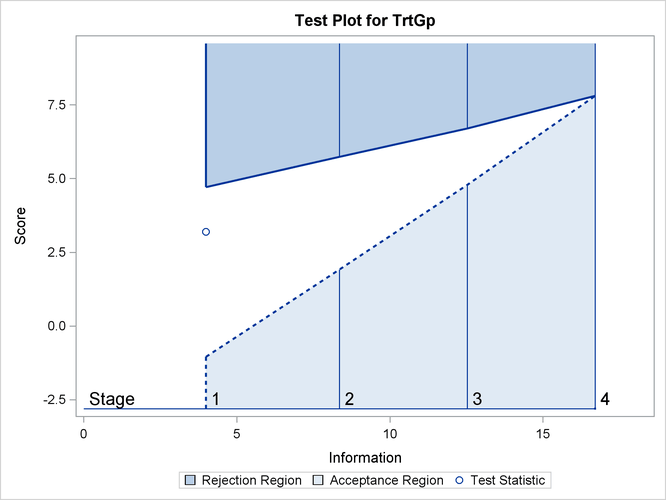Note that the input DATA= option can also be used for the test statistics. For example, the following statements create and display (in Output 84.6.13) the data set for the log-rank statistic and its associated standard error after the LIFETEST procedure. Since the log-rank statistic is a score statistic, the corresponding information level is the variance of the statistic.

proc lifetest data=Surv_1;
time Weeks*Event(0);
test TrtGp;
ods output logunichisq=Parms_Surv1a;
run;

data Parms_Surv1a;
set Parms_Surv1a(rename=(Statistic=TrtGp));
keep _Scale_ _Stage_ _Info_ TrtGp;
_Scale_='Score';
_Stage_= 1;
_Info_= StdErr * StdErr;
if Variable='TrtGp';
run;

proc print data=Parms_Surv1a;
title 'Statistics Computed at Stage 1';
run;


Output 84.6.13: Statistics Computed at Stage 1

 Statistics Computed at Stage 1

Obs TrtGp _Scale_ _Stage_ _Info_
1 3.2004 Score 1 3.99170

The following statements can be used to invoke the SEQTEST procedure to test for early stopping at stage 1:

ods graphics on;
proc seqtest Boundary=Bnd_Surv
Data(Testvar=TrtGp)=Parms_Surv1a
boundaryscale=score
;
ods output Test=Test_Surv1;
run;
ods graphics off;


The following statements use the LIFETEST procedure to compute the log-rank statistic and its associated standard error at stage 2:

proc lifetest data=Surv_2;
time Weeks*Event(0);
test TrtGp;
ods output logunichisq=Parms_Surv2;
run;


The following statements create and display (in Output 84.6.14) the data set for the log-rank statistic and its associated standard error for each of the first two stages:

data Parms_Surv2;
set Parms_Surv2 (rename=(Statistic=Estimate));
if Variable='TrtGp';
_Scale_='Score';
_Stage_= 2;
keep Variable _Scale_ _Stage_ StdErr Estimate;
run;

proc print data=Parms_Surv2;
title 'Statistics Computed at Stage 2';
run;


Output 84.6.14: Statistics Computed at Stage 2

 Statistics Computed at Stage 2

Obs Variable Estimate StdErr _Scale_ _Stage_
1 TrtGp 7.3136 2.9489 Score 2

The following statements invoke the SEQTEST procedure to test for early stopping at stage 2:

ods graphics on;
proc seqtest Boundary=Test_Surv1
Parms(Testvar=TrtGp)=Parms_Surv2
boundaryscale=score
citype=lower
;
ods output Test=Test_Surv2;
run;
ods graphics off;


The BOUNDARY= option specifies the input data set that provides the boundary information for the trial at stage 2, which was generated by the SEQTEST procedure at the previous stage. The PARMS= option specifies the input data set that contains the test statistic and its associated standard error at stage 2, and the TESTVAR= option identifies the test variable in the data set. The INFOADJ=NONE option maintains the information level for stage 3 at the value provided in the BOUNDARY= data set.

The ODS OUTPUT statement with the TEST=TEST_SURV2 option creates an output data set named TEST_SURV2 which contains the updated boundary information for the test at stage 2. The data set also provides the boundary information that is needed for the group sequential test at the next stage if the trial does not stop at the current stage.

The Test Information table in Output 84.6.15 displays the boundary values for the test statistic. The test statistic 7.31365 is larger than the corresponding upperboundary 5.79886, so the study stops and rejects the null hypothesis. That is, there is evidence of reduction in hazard rate for the new treatment.

Output 84.6.15: Sequential Tests

The SEQTEST Procedure

Test Information (Score Scale)
Null Reference = 0
_Stage_   Alternative Boundary Values Test
Information Level Reference Upper TrtGp
Proportion Actual Upper Beta Alpha Estimate Action
1 0.2389 3.991698 2.76685 -1.03860 4.71422 3.20040 Continue
2 0.5205 8.696125 6.02772 2.17045 5.79332 7.31365 Reject Null
3 0.7500 12.52968 8.68495 4.76306 6.72915 .
4 1.0000 16.70624 11.57993 7.81287 7.81287 .

With ODS Graphics enabled, the Test Plot displays boundary values of the design and the test statistic at the first two stages, as shown in Output 84.6.16. It also shows that the test statistic is in the Rejection Region above the upperboundary value at stage 2.

Output 84.6.16: Sequential Test Plot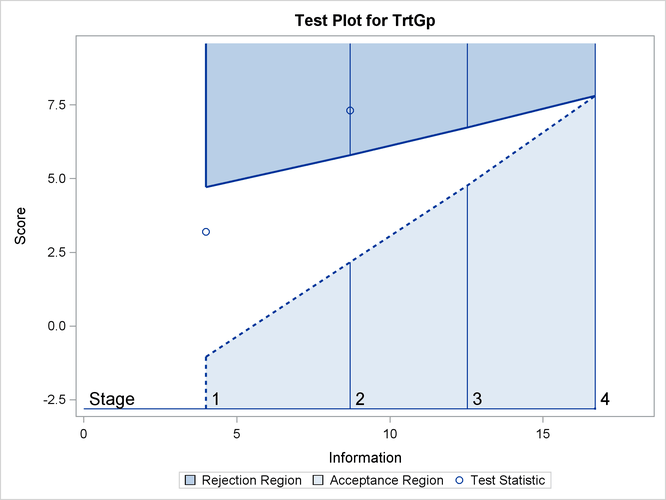After the stopping of a trial, the Parameter Estimates table in Output 84.6.17 displays the stopping stage, parameter estimate, unbiased median estimate, confidence limits, and p-value under the null hypothesis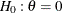.

Output 84.6.17: Parameter Estimates

Parameter Estimates
Stagewise Ordering
Parameter Stopping
Stage
MLE p-Value for
H0:Parm=0
Median
Estimate
Lower 95% CL
TrtGp 2 0.841024 0.0139 0.810329 0.21615

As expected, the p-value 0.0139 is significant at the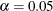level and the lower 95% confidence limit is larger than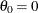. The p-value, unbiased median estimate, and lower confidence limit depend on the ordering of the sample space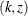, where k is the stage number and z is the standardized Z statistic. With the specified stagewise ordering, the p-value is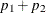, where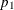is thespending at stage 1,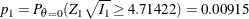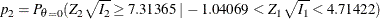where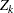is a standardized normal variate and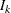is the information level at stage k for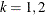.